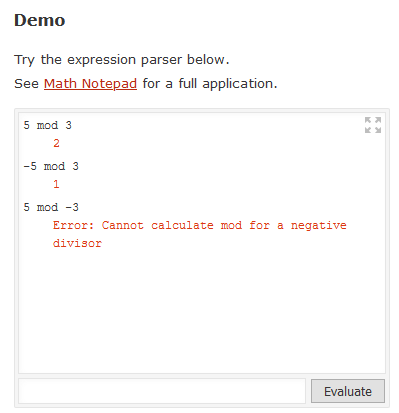{{ message }}
/ mathjs Public

# Modulo operation for negative values#1964

Closed
opened this issue Sep 11, 2020 · 6 comments
Closed

# Modulo operation for negative values#1964

opened this issue Sep 11, 2020 · 6 comments
Labels

###ovk commented Sep 11, 2020

 I noticed that `A mod N` (or `mod(A, N)`) operation in MathJS doesn't return expected values ("expected" in terms of modular arithmetic) for negative values of `A`. For example, `-5 mod 3` returns `-2` while the correct result should be `1` (example on wolframalpha). In addition, `mod` also works for negative values of `N` while I think modulo operation is only defined for `N > 1`. Here is an example of what MathJS returns (you can see it on clcalc that uses MathJS): `5 mod 3 = 2` `-5 mod 3 = -2` `5 mod -3 = 2` My guess is that such behaviour is the result of how `%` operator behaves in Javascript (example). However, while some programming languages like JS, or C/C++ (example) produce the same result (although, my understanding is that for C/C++ the result in case of negative `A` is implementation defined), some others, like Python, give different answer (example). I'm not sure what is the best way to address that. I think either MathJS `mod` operation should be fixed to behave like proper modulo operation, or the documentation/help should be changed to reflect the fact that it emulates what JavaScript `%` operator does, and is not proper modulo in terms of modular arithmetic. The text was updated successfully, but these errors were encountered:

###josdejong commented Sep 12, 2020 • edited

 Thanks for your inputs. If you try out those examples in the small demo calculator on https://mathjs.org/, it will return the outputs that you expect:I'm not sure what is going on in clcalc, it seems to be using the latest version of mathjs. Maybe it has loaded the buildin JavaScript mod function instead or so?

###ovk commented Sep 13, 2020

 Thanks for the pointers, @josdejong , I should've tried it myself before posting. I tracked down what the differences are and here is a minimal reproducible example: ```math.config({ 'number': 'BigNumber' }); // Prints: -2 console.log(math.evaluate('-5 mod 3').toString());``` If you comment out the first line, then it returns the correct results (`1`). On clcalc I always use BigNumber which is why `mod` always appears "broken". Looking at MathJS code for `mod` it looks like in the case of `BigNumber` it just invokes `mod` from the bignumber library. In the library itself there is `MODULO_MODE` (https://github.com/MikeMcl/bignumber.js/blob/master/bignumber.js#L144) which perhaps should be set to `EUCLID` to be consistent with MathJS `mod` when operating on Numbers?

###josdejong commented Sep 13, 2020

 Ahhh, that makes sense. Yes, then we should change the `MODULO_MODE` of the library (I wasn't aware there was a setting for this). Anyone interested in working this out in a PR and adding a few unit tests?

###ovk commented Sep 14, 2020

 I also noticed the same issue with fractions: ``````mod(fraction("-5"), fraction("3")) -2/1 `````` Unless somebody else volunteers, I'll work on a PR in a week or so (once I'm back to my computer).

###josdejong commented Sep 14, 2020

 Thanks @ovk , would be great if you could pick this up 👍

###josdejong commented Sep 26, 2020

 Fix is published now in `v7.3.0` 🎉